Definitions of Acids and Bases, and the Role of Water

Definitions of Acids and Bases
and the Role of Water

Properties of Acids and Bases According to Boyle

In 1661 Robert Boyle summarized the properties of acids as follows.

1. Acids have a sour taste.

2. Acids are corrosive.

3. Acids change the color of certain vegetable dyes, such as litmus, from blue to red.

4. Acids lose their acidity when they are combined with alkalies.

The name "acid" comes from the Latin acidus, which means "sour," and refers to the sharp odor and sour taste of many acids.

Examples: Vinegar tastes sour because it is a dilute solution of acetic acid in water. Lemon juice tastes sour because it contains citric acid. Milk turns sour when it spoils because lactic acid is formed, and the unpleasant, sour odor of rotten meat or butter can be attributed to compounds such as butyric acid that form when fat spoils.

In 1661 Boyle summarized the properties of alkalies as follows.

• Alkalies feel slippery.
• Alkalies change the color of litmus from red to blue.
• Alkalies become less alkaline when they are combined with acids.

In essence, Boyle defined alkalies as substances that consume, or neutralize, acids. Acids lose their characteristic sour taste and ability to dissolve metals when they are mixed with alkalies. Alkalies even reverse the change in color that occurs when litmus comes in contact with an acid. Eventually alkalies became known as bases because they serve as the "base" for making certain salts.In 1884 Svante Arrhenius suggested that salts such as NaCl dissociate when they dissolve in water to give particles he called ions.

 H2O NaCl(s)Na+(aq) + Cl-(aq)

Three years later Arrhenius extended this theory by suggesting that acids are neutral compounds that ionize when they dissolve in water to give H+ ions and a corresponding negative ion. According to his theory, hydrogen chloride is an acid because it ionizes when it dissolves in water to give hydrogen (H+) and chloride (Cl-) ions as shown in the figure below.

 H2O HCl(g)H+(aq) + Cl-(aq)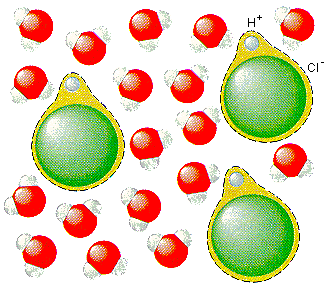Arrhenius argued that bases are neutral compounds that either dissociate or ionize in water to give OH- ions and a positive ion. NaOH is an Arrhenius base because it dissociates in water to give the hydroxide (OH-) and sodium (Na+) ions.

 H2O NaOH(s)Na+(aq) + OH-(aq)

An Arrhenius acid is therefore any substance that ionizes when it dissolves in water to give the H+, or hydrogen, ion.

An Arrhenius base is any substance that gives the OH-, or hydroxide, ion when it dissolves in water.

Arrhenius acids include compounds such as HCl, HCN, and H2SO4 that ionize in water to give the H+ ion. Arrhenius bases include ionic compounds that contain the OH- ion, such as NaOH, KOH, and Ca(OH)2.

This theory explains why acids have similar properties: The characteristic properties of acids result from the presence of the H+ ion generated when an acid dissolves in water. It also explains why acids neutralize bases and vice versa. Acids provide the H+ ion; bases provide the OH- ion; and these ions combine to form water.

H+(aq) + OH-(aq)H2O(l)

The Arrhenius theory has several disadvantages.

• It can be applied only to reactions that occur in water because it defines acids and bases in terms of what happens when compounds dissolve in water.
• It doesn't explain why some compounds in which hydrogen has an oxidation number of +1 (such as HCl) dissolve in water to give acidic solutions, whereas others (such as CH4) do not.
• Only the compounds that contain the OH- ion can be classified as Arrhenius bases. The Arrhenius theory can't explain why other compounds (such as Na2CO3) have the characteristic properties of bases.The Role of H+ and OH- Ions In the Chemistry of Aqueous Solutions

Becuase oxygen (EN = 3.44) is much more electronegative than hydrogen (EN = 2.20), the electrons in the HO bonds in water aren't shared equally by the hydrogen and oxygen atoms. These electrons are drawn toward the oxygen atom in the center of the molecule and away from the hydrogen atoms on either end. As a result, the water molecule is polar. The oxygen atom carries a partial negative charge (-), and the hydrogen atoms carry a partial positive charge (+).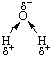When they dissociate to form ions, water molecules therefore form a positively charged H+ ion and a negatively charged OH- ion.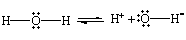The opposite reaction can also occurH+ ions can combine with OH- ions to form neutral water molecules.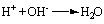The fact that water molecules dissociate to form H+ and OH- ions, which can then recombine to form water molecules, is indicated by the following equation.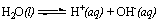To What Extent Does Water Dissociate to Form Ions?

At 25C, the density of water is 0.9971 g/cm3, or 0.9971 g/mL. The concentration of water is therefore 55.35 molar.The concentration of the H+ and OH- ions formed by the dissociation of neutral H2O molecules at this temperature is only 1.0 x 10-7 mol/L. The ratio of the concentration of the H+ (or OH-) ion to the concentration of the neutral H2O molecules is therefore 1.8 x 10-9.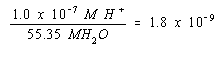In other words, only about 2 parts per billion (ppb) of the water molecules dissociate into ions at room temperature. The figure below shows a model of 20 water molecules, one of which has dissociated to form a pair of H+ and OH- ions. If this illustration was a very-high-resolution photograph of the structure of water, we would encounter a pair of H+and OH- ions on the average of only once for every 25 million such photographs.The Operational Definition of Acids and Bases

The fact that water dissociates to form H+ and OH- ions in a reversible reaction is the basis for an operational definition of acids and bases that is more powerful than the definitions proposed by Arrhenius. In an operational sense, an acid is any substance that increases the concentration of the H+ ion when it dissolves in water. A base is any substance that increases the concentration of the OH- ion when it dissolves in water.

These definitions tie the theory of acids and bases to a simple laboratory test for acids and bases. To decide whether a compound is an acid or a base we dissolve it in water and test the solution to see whether the H+ or OH- ion concentration has increased.The properties of acids and bases result from differences between the chemistry of metals and nonmetals, as can be seen from the chemistry of these classes of compounds: hydrogne, oxides, and hydroxides.

Compounds that contain hydrogen bound to a nonmetal are called nonmetal hydrides. Because they contain hydrogen in the +1 oxidation state, these compounds can act as a source of the H+ ion in water.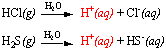Metal hydrides, on the other hand, contain hydrogen bound to a metal. Because these compounds contain hydrogen in a -1 oxidation state, they dissociate in water to give the H- (or hydride) ion.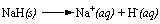The H- ion, with its pair of valence electrons, can abstract an H+ ion from a water molecule.Since removing H+ ions from water molecules is one way to increase the OH- ion concentration in a solution, metal hydrides are bases.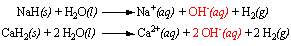A similar pattern can be found in the chemistry of the oxides formed by metals and nonmetals. Nonmetal oxides dissolve in water to form acids. CO2 dissolves in water to give carbonic acid, SO3 gives sulfuric acid, and P4O10 reacts with water to give phosphoric acid.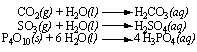Metal oxides, on the other hand, are bases. Metal oxides formally contain the O2- ion, which reacts with water to give a pair of OH- ions.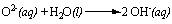Metal oxides therefore fit the operational definition of a base.We see the same pattern in the chemistry of compounds that contain theOH, or hydroxide, group. Metal hydroxides, such as LiOH, NaOH, KOH, and Ca(OH)2, are bases.Nonmetal hydroxides, such as hypochlorous acid (HOCl), are acids.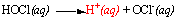The table below summarizes the trends observed in these three categories of compounds. Metal hydrides, metal oxides, and metal hydroxides are bases. Nonmetal hydrides, nonmetal oxides, and nonmetal hydroxides are acids.

Typical Acids and Bases

 Acids Bases Non-metal Hydrides HF, HCl, HBr, HCN, HSCN, H2S Metal Hydrides HI, LiH, NaH, KH, MgH2, CaH2 Non-metal Oxides CO2, SO2, SO3, NO2, P4O10 Metal Oxides Li2O, Na2O, K2O, MgO, CaO Non-metal Hydroxides HOCl, HONO2, O2S(OH)2, OP(OH)3 Metal Hydroxides LiOH, NaOH, KOH, Ca(OH)2, Ba(OH)2

The acidic hydrogen atoms in the non-metal hydroxides in the table above aren't bound to the nitrogen, sulfur, or phosphorus atoms. In each of these compounds, the acidic hydrogen is attached to an oxygen atom. These compounds are therefore all examples of oxyacids.

Skeleton structures for eight oxyacids are given in the figure below. As a general rule, acids that contain oxygen have skeleton structures in which the acidic hydrogens are attached to oxygen atoms.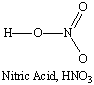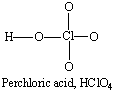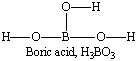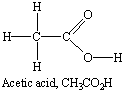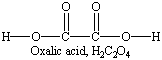Practice Problem 1:Use Lewis structures to classify the following acids as either nonmetal hydrides (XH) or nonmetal hydroxides (XOH). (a) HCN (b) HNO3 (c) H2C2O4 (d) CH3CO2H Click here to check your answer to Practice Problem 1Why are Metal Hydroxides Bases and Nonmetal Hydroxides Acids?

To understand why nonmetal hydroxides are acids and metal hydroxides are bases, we have to look at the electronegativities of the atoms in these compounds. Let's start with a typical metal hydroxide: sodium hydroxideThe difference between the electronegativities of sodium and oxygen is very large (EN = 2.5). As a result, the electrons in the NaO bond are not shared equallythese electrons are drawn toward the more electronegative oxygen atom. NaOH therefore dissociates to give Na+ and OH- ions when it dissolves in water.We get a very different pattern when we apply the same procedure to hypochlorous acid, HOCl, a typical nonmetal hydroxide.Here, the difference between the electronegativities of the chlorine and oxygen atoms is small (EN = 0.28). As a result, the electrons in the ClO bond are shared more or less equally by the two atoms. The OH bond, on the other hand, is polar (EN = 1.24)the electrons in this bond are drawn toward the more electronegative oxygen atom. When this molecule ionizes, the electrons in the O-H bond remain with the oxygen atom, and OCl- and H+ ions are formed.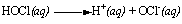There is no abrupt change from metal to nonmetal across a row or down a column of the periodic table. We should therefore expect to find compounds that lie between the extremes of metal and nonmetal oxides, or metal and nonmetal hydroxides. These compounds, such as Al2O3 and Al(OH)3, are called amphoteric (literally, "either or both") because they can act as either acids or bases. Al(OH)3, for example, acts as an acid when it reacts with a base.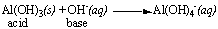Conversely, it acts as a base when it reacts with an acid.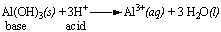The Brnsted Definition of Acids and Bases

The Brnsted, or Brnsted-Lowry, model is based on a simple assumption: Acids donate H+ ions to another ion or molecule, which acts as a base. The dissociation of water, for example, involves the transfer of an H+ ion from one water molecule to another to form H3O+ and OH- ions.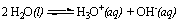According to this model, HCl doesn't dissociate in water to form H+ and Cl+ ions. Instead, an H+ ion is transferred from HCl to a water molecule to form H3O+ and Cl- ions, as shown in the figure below.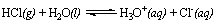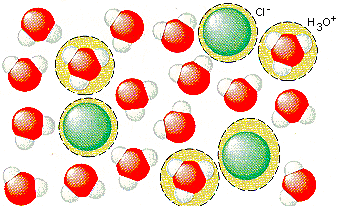Because it is a proton, an H+ ion is several orders of magnitude smaller than the smallest atom. As a result, the charge on an isolated H+ ion is distributed over such a small amount of space that this H+ ion is attracted toward any source of negative charge that exists in the solution. Thus, the instant that an H+ ion is created in an aqueous solution, it bonds to a water molecule. The Brnsted model, in which H+ ions are transferred from one ion or molecule to another, therefore makes more sense than the Arrhenius theory, which assumes that H+ ions exist in aqueous solution.

Even the Brnsted model is naive. Each H+ ion that an acid donates to water is actually bound to four neighboring water molecules, as shown in the figure below.A more realistic formula for the substance produced when an acid loses an H+ ion is therefore H(H2O)4+, or H9O4+. For all practical purposes, however, this substance can be represented as the H3O+ ion.

The reaction between HCl and water provides the basis for understanding the definitions of a Brnsted acid and a Brnsted base. According to this theory, an H+ ion is transferred from an HCl molecule to a water molecule when HCl dissociates in water.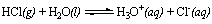HCl acts as an H+-ion donor in this reaction, and H2O acts as an H+ ion-acceptor. A Brnsted acid is therefore any substance (such as HCl) that can donate an H+ ion to a base. A Brnsted base is any substance (such as H2O) that can accept an H+ ion from an acid.

There are two ways of naming the H+ ion. Some chemists call it a hydrogen ion; others call it a proton. As a result, Brnsted acids are known as either hydrogen-ion donors or proton donors. Brnsted bases are hydrogen-ion acceptors or proton acceptors.

From the perspective of the Brnsted model, reactions between acids and bases always involve the transfer of an H+ ion from a proton donor to a proton acceptor. Acids can be neutral molecules.They can also be positive ions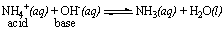or negative ions.The Brnsted theory therefore expands the number of potential acids. It also allows us to decide which compounds are acids from their chemical formulas. Any compound that contains hydrogen with an oxidation number of +1 can be an acid. Brnsted acids include HCl, H2S, H2CO3, H2PtF6, NH4+, HSO4-, and HMnO4.

Brnsted bases can be identified from their Lewis structures. According to the Brnsted model, a base is any ion or molecule that can accept a proton. To understand the implications of this definition, look at how the prototypical base, the OH- ion, accepts a proton.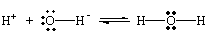The only way to accept an H+ ion is to form a covalent bond to it. In order to form a covalent bond to an H+ ion that has no valence electrons, the base must provide both of the electrons needed to form the bond. Thus, only compounds that have pairs of nonbonding valence electrons can act as H+-ion acceptors, or Brnsted bases.

The following compounds, for example, can all act as Brnsted bases because they all contain nonbonding pairs of electrons.The Brnsted model expands the list of potential bases to include any ion or molecule that contains one or more pairs of nonbonding valence electrons. The Brnsted definition of a base applies to so many ions and molecules that it is almost easier to count substances, such as the following, that can't be Brnsted bases because they don't have pairs of nonbonding valence electrons.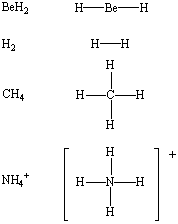Practice Problem 2:Which of the following compounds can be Brnsted acids? Which can be Brnsted bases? (a) H2O (b) NH3 (c) HSO4- (d) OH- Click here to check your answer to Practice Problem 2The Role of Water in the Brnsted Theory

The Brnsted theory explains water's role in acid-base reactions.

• Water dissociates to form ions by transferring an H+ ion from one molecule acting as an acid to another molecule acting as a base.
 H2O(l) + H2O(l)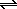H3O+(aq) + OH-(aq) acid base
• Acids react with water by donating an H+ ion to a neutral water molecule to form the H3O+ ion.
 HCl(g) + H2O(l)H3O+(aq) + Cl-(aq) acid base
• Bases react with water by accepting an H+ ion from a water molecule to form the OH- ion.
 NH3(aq) + H2O(l)NH4+(aq) + OH-(aq) base acid
• Water molecules can act as intermediates in acid-base reactions by gaining H+ ions from the acid
 HCl(g) + H2O(l)H3O+(aq) + Cl-(aq)

and then losing these H+ ions to the base.

 NH3(aq) + H3O+(aq)NH4+(aq) + H2O(l)

The Brnsted model can be extended to acid-base reactions in other solvents. For example, there is a small tendency in liquid ammonia for an H+ ion to be transferred from one NH3 molecule to another to form the NH4+ and NH2- ions.

 2 NH3NH4+ + NH2-

By analogy to the chemistry of aqueous solutions, we conclude that acids in liquid ammonia include any source of the NH4+ ion and that bases include any source of the NH2- ion.

The Brnsted model can even be extended to reactions that don't occur in solution. A classic example of a gas-phase acid-base reaction is encountered when open containers of concentrated hydrochloric acid and aqueous ammonia are held next to each other. A white cloud of ammonium chloride soon forms as the HCl gas that escapes from one solution reacts with the NH3 gas from the other.

 HCl(g) + NH3(g)NH4Cl(s)

This reaction involves the transfer of an H+ ion from HCl to NH3 and is therefore a Brnsted acid-base reaction, even though it occurs in the gas phase.